Debadri Sengupta — December 7, 2020

This article was published as a part of the Data Science Blogathon.

## BackgroundI won’t be lying if I assert that every developer/engineer/student has used the website Stack Overflow more than once in their journey. Widely considered as one of the largest and more trusted websites for developers to learn and share their knowledge, the website presently hosts in excess of 10,000,000 questions. In this post, we try to predict the question tags based on the question text asked on Stack Overflow. The most common question tags on Stack Overflow include Java, JavaScript, C#, PHP, Android amongst others.

Proper prediction of the tags is important to ensure that the questions are suggested to users having substantial experience in answers related to the topic suggested.

## Data

The data was collected from the Keyword Extraction competition by Facebook on Kaggle (https://www.kaggle.com/c/facebook-recruiting-iii-keyword-extraction/data).

The training data consists of 4 columns- Id, Title, Body, Tags. The body consists of a lot of computer code- an issue to be addressed early.

## Constraints

As with every information retrieval model, high precision and high recall is a must. An incorrect label affects precision while a missed label affects recall. Both hamper user experience on the site. Latency requirements are not stringent.

## The prediction problem

Our problem is essentially a multi-label classification problem where each of the question tags in Stack Overflow website is the label and their prediction essentially is not mutually exclusive.

The evaluation metric to be used is the micro-averaged F1-score which beautifully inculcates both precision and recall simultaneously.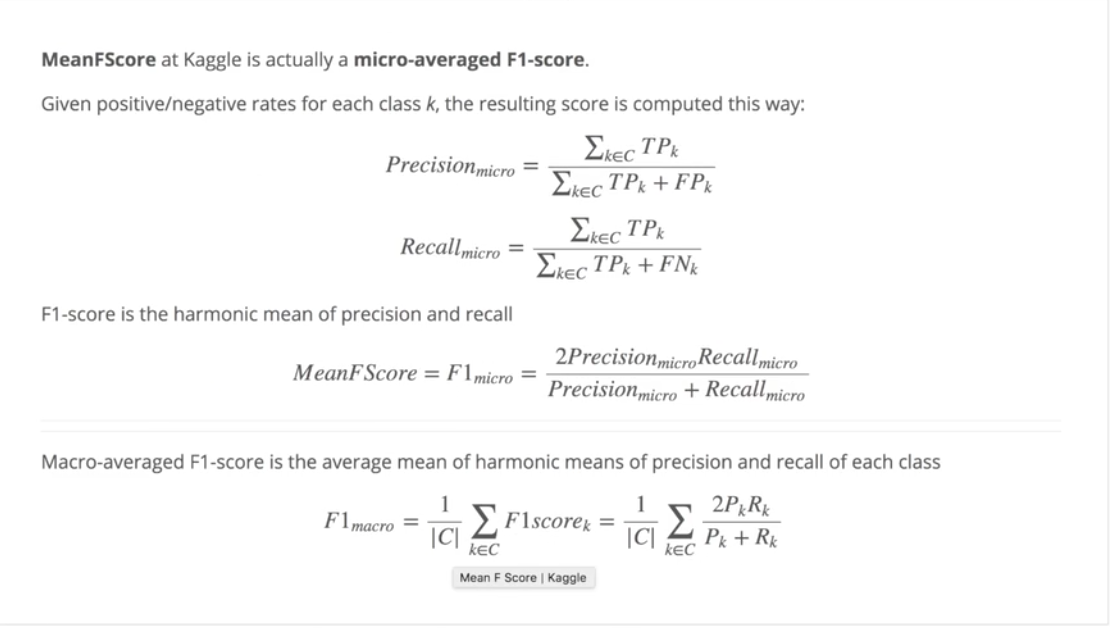Because the micro F1-score includes the number of true positives, false positives and false negatives of a particular class, it also inherently weighs each class by the frequency of data points for it. However, the macro F1-score is free from such bias. It is a simple average function.

We use the micro F1-score to account for the unequal size of our classes.

## Exploratory Data Analysis

The data is loaded from the CSV files to an SQLite database. Duplicate questions removal is done through SQL queries. The original number of rows in the database was 6034196. The new database, post deduplication, consists of 4206314 rows.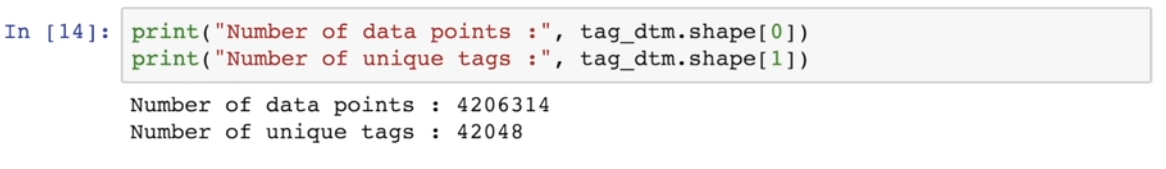```#Code to get the bag of words vocabulary for the tags
tags = vectorizer.get_feature_names()```

Let us take a look at some alphabetically sorted tags and their counts.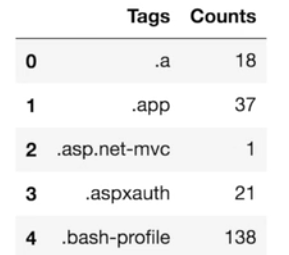Now, we sort the tags in decreasing order of the number of occurrences and then plot the distribution.

```plt.plot(tag_counts)
plt.title(“Distribution of number of times tag appeared questions”)
plt.grid()plt.xlabel(“Tag number”)
plt.ylabel(“Number of times tag appeared”)
plt.show()```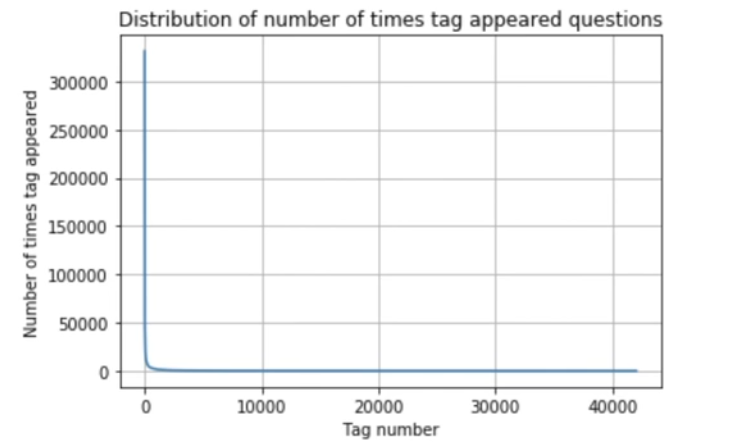Noticeably, the distribution is highly skewed with the most frequent tag occurring more than 3 million times, and the rarer ones occurring around once.

We need to zoom into the data to get a better understanding of the distribution. We consider the top 100 tags now.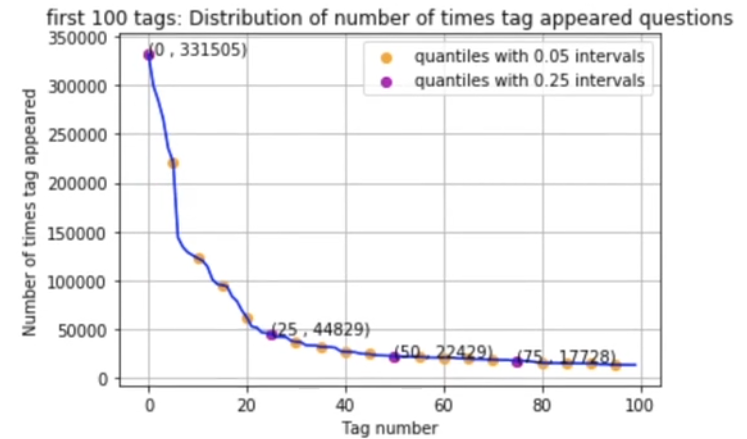The drops in the quantiles are still significant. The 25th percentile consists of barely 15 percent of the 0 percentile values. The numbers get lower with drop-in quantiles.

In fact, just the top 14 tags have been used more than 1 million times. The most frequent tag (C#) is used 331505 times.

The enormous variation in the frequency of tags justifies the micro-averaged F1-score as the appropriate metric.

We now plot the number of tags per question.

```sns.countplot(tag_quest_count, palette='gist_rainbow')
plt.title("Number of tags in the questions ")
plt.xlabel("Number of Tags")
plt.ylabel("Number of questions")
plt.show()```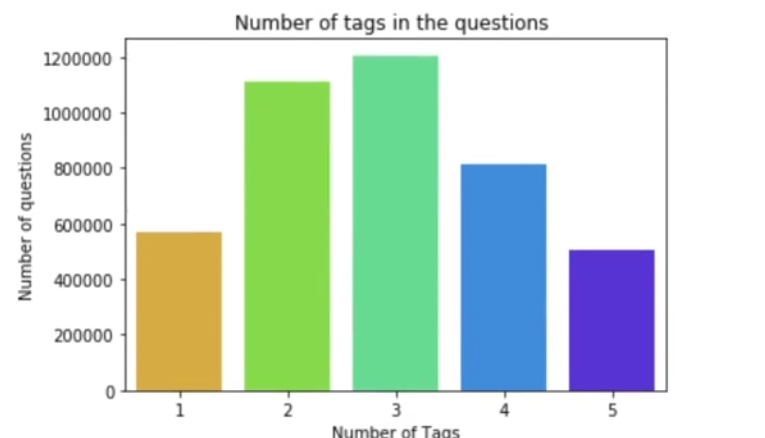Most questions have 2–3 tags the average being around 2.9. The range of tags per question is from 1 to 5.

We now plot the Word Cloud for the tags. The font size of each word is proportional to its frequency of occurrence.

```tup = dict(result.items())#Initializing WordCloud using frequencies of tags.
wordcloud = WordCloud( background_color=’black’,width=1600,height=800,).generate_from_frequencies(tup)
fig = plt.figure(figsize=(30,20))
plt.imshow(wordcloud)plt.axis(‘off’)
fig.savefig(“tag.png”)
plt.show()```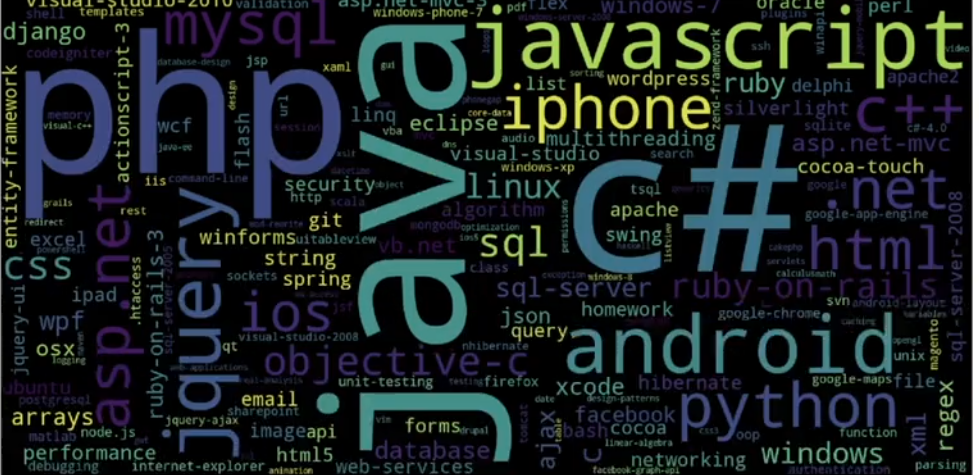Let’s take a look at the top 20 tags.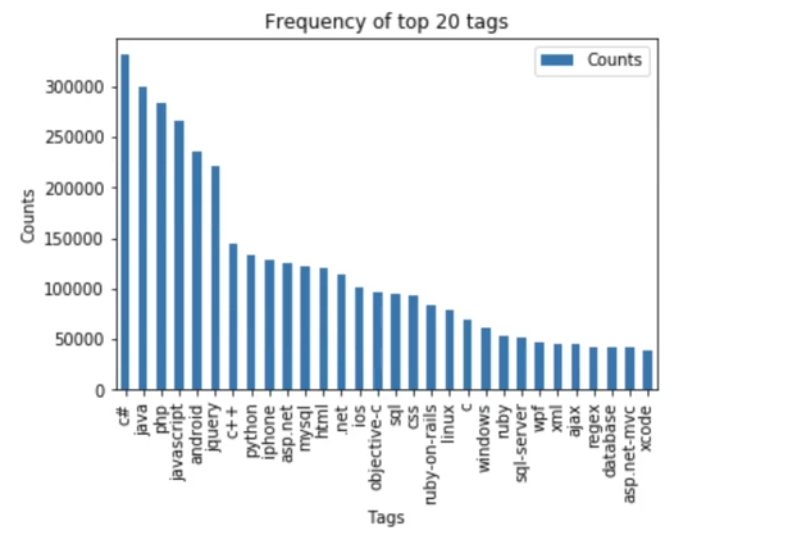Most of the top tags are associated with programming languages and platforms.

## Text preprocessing

Due to computational limitations, 1 million data points have been sampled out of the 4 million.

Let’s take a look at an example data point.

```Title:  Implementing Boundary Value Analysis of Software Testing in a C++ program?
Body :#include<
iostream>n
#include<
stdlib.h>nn
using namespace std;nn
int main()n
{n
int n,a[n],x,c,u[n],m[n],e[n];n
cout<<"Enter the number of variables";n         cin>>n;nn
cout<<"Enter the Lower, and Upper Limits of the variables";n
for(int y=1; y<n+1; y++)n
{n
cin>>m[y];n
cin>>u[y];n
}n
for(x=1; x<n+1; x++)n
{n
a[x] = (m[x] + u[x])/2;n
}n
c=(n*4)-4;n
for(int a1=1; a1<n+1; a1++)n
{nn
e[a1] = m[a1];n
e[a1] = m[a1]+1;n
e[a1] = u[a1]-1;n
e[a1] = u[a1];n
}n
for(int i=1; i<n+1; i++)n
{n
for(int l=1; l<=i; l++)n
{n
if(l!=1)n
{n
cout<<a[l]<<"\t";n
}n
}n
for(int j=0; j<4; j++)n
{n
cout<<e[i][j];n
for(int k=0; k<n-(i+1); k++)n
{n
cout<<a[k]<<"\t";n
}n
cout<<"\n";n
}n
}    nn
system("PAUSE");n
return 0;    n
}nnn<p>The answer should come in the form of a table like</p>nn
<pre><code>
1            50              50n
2            50              50n
99           50              50n
100          50              50n
50           1               50n
50           2               50n
50           99              50n
50           100             50n
50           50              1n
50           50              2n
50           50              99n
50           50              100n
</code></pre>nn
<p>if the no of inputs is 3 and their ranges aren
1,100n
1,100n
1,100n
(could be varied too)</p>nn
<p>The output is not coming,can anyone correct the code or tell me what's wrong?</p>n'Tags : 'c++ c'```

The following steps were taken for the preprocessing-

1. The code snippets were separated from the remaining text in the body of the question.
2. Special characters were removed from the title and the body (excluding the code) using regular expressions.
3. Stop-words were removed. (except ‘C’).
4. HTML tags (‘<something>’) were removed.
5. Conversion of words to lowercase followed by stemming by Snowball Stemmer.

This is how some question bodies look after processing.

`('ef code first defin one mani relationship differ key troubl defin one zero mani relationship entiti ef object model look like use fluent api object composit pk defin batch id batch detail id use fluent api object composit pk defin batch detail id compani id map exist databas tpt basic idea submittedtransact zero mani submittedsplittransact associ navig realli need one way submittedtransact submittedsplittransact need dbcontext class onmodelcr overrid map class lazi load occur submittedtransact submittedsplittransact help would much appreci edit taken advic made follow chang dbcontext class ad follow onmodelcr overrid must miss someth get follow except thrown submittedtransact key batch id batch detail id zero one mani submittedsplittransact key batch detail id compani id rather assum convent creat relationship two object configur requir sinc obvious wrong',) ---------------------------------------------------------------------------------------------------- ('explan new statement review section c code came accross statement block come accross new oper use way someon explain new call way',) ---------------------------------------------------------------------------------------------------- ('error function notat function solv logic riddl iloczyni list structur list possibl candid solut list possibl coordin matrix wan na choos one candid compar possibl candid element equal wan na delet coordin call function skasuj look like ni knowledg haskel cant see what wrong',) ---------------------------------------------------------------------------------------------------- ('step plan move one isp anoth one work busi plan switch isp realli soon need chang lot inform dns wan wan wifi question guy help mayb peopl plan correct chang current isp new one first dns know receiv new ip isp major chang need take consider exchang server owa vpn two site link wireless connect km away citrix server vmware exchang domain control link place import server crucial step inform need know avoid downtim busi regard ndavid',) ---------------------------------------------------------------------------------------------------- ('use ef migrat creat databas googl migrat tutori af first run applic creat databas ef enabl migrat way creat databas migrat rune applic tri',) ---------------------------------------------------------------------------------------------------- ('magento unit test problem magento site recent look way check integr magento site given point unit test jump one method would assum would big job write whole lot test check everyth site work anyon involv unit test magento advis follow possibl test whole site custom modul nis exampl test would amaz given site heavili link databas would nbe possibl fulli test site without disturb databas better way automaticlli check integr magento site say integr realli mean fault site ship payment etc work correct',) ---------------------------------------------------------------------------------------------------- ('find network devic without bonjour write mac applic need discov mac pcs iphon ipad connect wifi network bonjour seem reason choic turn problem mani type router mine exampl work block bonjour servic need find ip devic tri connect applic specif port determin process run best approach accomplish task without violat app store sandbox',) ---------------------------------------------------------------------------------------------------- ('send multipl row mysql databas want send user mysql databas column user skill time nnow want abl add one row user differ time etc would code send databas nthen use help schema',) ---------------------------------------------------------------------------------------------------- ('insert data mysql php powerpoint event powerpoint present run continu way updat slide present automat data mysql databas websit',) ----------------------------------------------------------------------------------------------------`

## Modelling-

It is crucial to propose this multi-label classification problem as a binary classification for us to apply various ML models. One vs Rest Classification will be too slow on 42000 labels. Classifier chains’ use is pertinent as the tags themselves may be correlated. For example, the presence of ‘C++’ may also trigger the presence of ‘pointers’.

Label power-sets may lead to the creation of a massive number of classes. Adoption of existing algorithms for multi-label classification is another approach More information on this topic can be found on this link.

Due to computation limitations, we cannot use all the tag labels as classes. We need to choose the top tags as per occurrence frequency for a ‘partial coverage’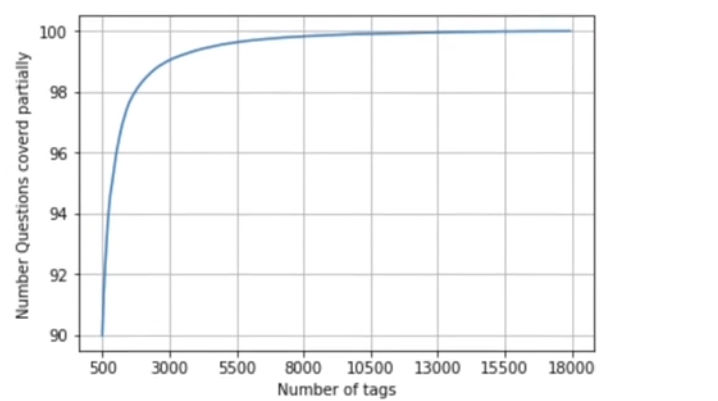Percentage of questions covered vs no of tags

Only using the top 5500 tags makes us cover more than 99 percent of questions partially.

Train-test-split of 80–20 ratio is performed randomly.

```total_size=preprocessed_data.shapetrain_size=int(0.80*total_size)
x_test=preprocessed_data.tail(total_size — train_size)
y_train = multilabel_yx[0:train_size,:]
y_test = multilabel_yx[train_size:total_size,:]```

We now convert our pre-processed text data of the question into its Tf-Idf form.

```vectorizer = TfidfVectorizer(min_df=0.00009, max_features=200000, smooth_idf=True, norm="l2",
tokenizer = lambda x: x.split(), sublinear_tf=False, ngram_range=(1,3))
x_train_multilabel = vectorizer.fit_transform(x_train['question'])
x_test_multilabel = vectorizer.transform(x_test['question'])```

Our feature vector is 88244 dimensional while the label vector is 5500 dimensional. We run into a problem here. The feature vector obtained is a very sparse vector. ‘scikit-multilearn’ library tries to convert such large sparse vectors to dense ones which raise us a memory error. Hence we fail to use MLKNN (a multi-label algorithm for KNN) in this case.

We instead use One vs Rest Classifier. The algorithm trains 5500 classifiers separately and uses their results.

```classifier = OneVsRestClassifier(SGDClassifier(loss='log', alpha=0.00001, penalty='l1'), n_jobs=-1)
classifier.fit(x_train_multilabel, y_train)
predictions = classifier.predict(x_test_multilabel)
print("accuracy :",metrics.accuracy_score(y_test,predictions))
print("macro f1 score :",metrics.f1_score(y_test, predictions, average = 'macro'))
print("micro f1 scoore :",metrics.f1_score(y_test, predictions, average = 'micro'))
print("hamming loss :",metrics.hamming_loss(y_test,predictions))```

Here’s the precision-recall report for the first 10 labels-

As we observe, the metrics are relatively low. Hyperparameter tuning is restricted due to the large size of the dataset. The training takes around 8 hours on a mid-level laptop (i5 processor). Hence, we repeat the same procedure but now for 0.5 million data points and taking the top 500 labels (accounting for 90 percent partial coverage of questions). We also emphasize extra weight on the title of the question rather than the body.

As seen, the metrics have improved from the previous training. The recall remains low which increases our model’s chances of missing labels. Further tuning may be used to correct this issue.

Hope you found the article helpful!